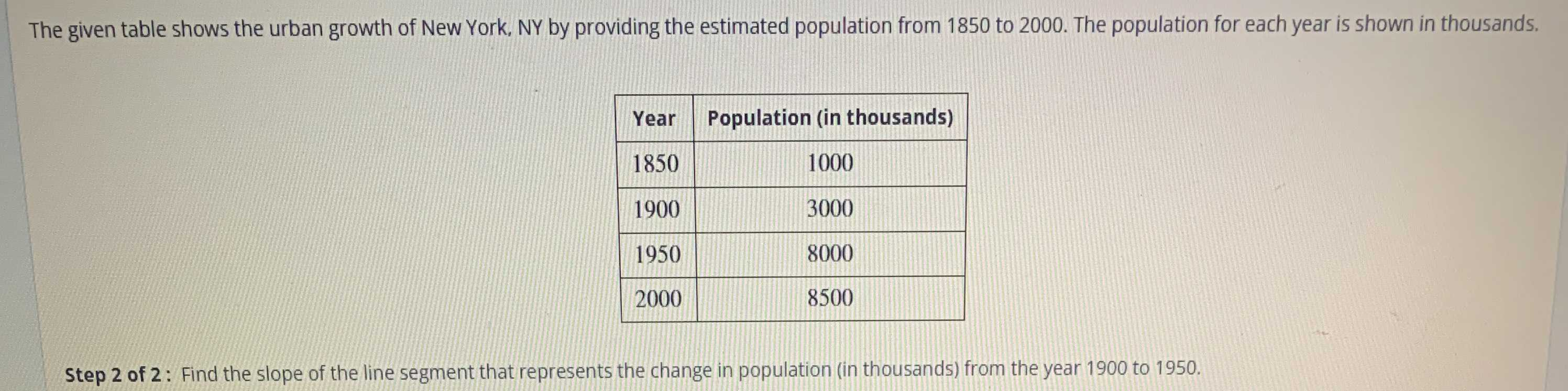### Still have math questions?

Algebra
QuestionThe given table shows the urban growth of New York, NY by providing the estimated population from $$1850$$ to $$2000$$ . The population for each year is shown in thousands.

Step $$2$$ of $$2$$ : Find the slope of the line segment that represents the change in population (in thousands) from the year $$1900$$ to $$1950 .$$